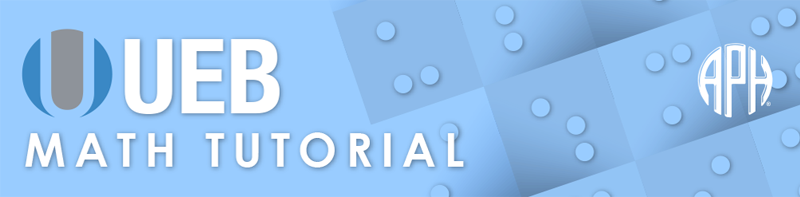Practice Problems -   -  Use 6 Dot Entry   Switch to Nemeth Tutorial

# Lesson 7.2: Set Notation: Union, Intersections and the Infinity Symbol

## Symbols

$\cup \phantom{\rule{.3em}{0ex}}\text{union}$
⠨⠖

$\cap \phantom{\rule{.3em}{0ex}}\text{intersection}$
⠨⠦

$\infty \phantom{\rule{.3em}{0ex}}\text{infinity symbol}$
⠼⠿

## Review

In set notation, each element of the set is listed and separated by a comma, with all of the elements between curly brackets or braces. The numeric indicator is required with any numeral that immediately follows a bracket or brace since both of these symbols terminate numeric mode. Sets are typically named using capital letters. A single letter other than A, I, or O requires a grade 1 indicator when it is standing alone. Follow print for spacing of symbols.

## Explanation

Set notation describes the relationship of one set to another. The union of a collection of sets is the set of all elements in the collection and is denoted by the union symbol in print, ∪. In braille the union symbol is formed with two cells, dots four six in the first cell and dots two three five in the second cell. The intersection of two sets is the set that contains all elements of one set that also belong to the other, but no other elements. The intersection is denoted by the intersection symbol, ∩. In braille, this symbol is made with two cells, dots four six in the first and dots two three six in the second. Follow print for use and spacing of the symbols.

The infinity symbol is often represented by a figure that looks like a numeral eight in the horizontal position in print. In braille, this symbol is made with two cells; the numeric prefix, dots three four five six in the first cell and a full cell, dots one two three four five six in the second.

### Example 1

$\infty +1=\infty$
⠼⠿⠐⠖⠼⠁⠀⠐⠶⠀⠼⠿

### Example 2

$-\infty ×\infty =-\infty$
⠐⠤⠼⠿⠐⠦⠼⠿⠀⠐⠶⠀⠐⠤⠼⠿

### Example 3

$59\phantom{\rule{.3em}{0ex}}\text{to}\phantom{\rule{.3em}{0ex}}\infty$
⠼⠑⠊⠀⠞⠕⠀⠼⠿

### Example 4

$\text{The line goes to}\phantom{\rule{.3em}{0ex}}\infty \text{.}$
⠠⠮⠀⠇⠔⠑⠀⠛⠕⠑⠎⠀⠞⠕⠀⠼⠿⠲

### Example 5

$\left[20,\infty \right)$
⠨⠣⠼⠃⠚⠂⠀⠼⠿⠐⠜

### Example 6

$\left(-\infty ,15\right]$
⠐⠣⠐⠤⠼⠿⠂⠀⠼⠁⠑⠨⠜

### Example 7

$A\cap B=\left\{3\right\}$
⠠⠁⠨⠦⠠⠃⠀⠐⠶⠀⠸⠣⠼⠉⠸⠜

### Example 8

$A\cup B=\left\{1,2,3\right\}$
⠠⠁⠨⠖⠠⠃⠀⠐⠶⠀⠸⠣⠼⠁⠂⠀⠼⠃⠂⠀⠼⠉⠸⠜

### Example 9

${B}^{\prime }=\left\{2,4,6\right\}$
⠠⠃⠰⠶⠀⠐⠶⠀⠸⠣⠼⠃⠂⠀⠼⠙⠂⠀⠼⠋⠸⠜

### Example 10

$A\cap B=\varnothing$
⠠⠁⠨⠦⠠⠃⠀⠐⠶⠀⠈⠚

### Example 11

${P}^{\prime }\cup {Q}^{\prime }={\left(P\cap Q\right)}^{\prime }$
⠰⠰⠰⠠⠏⠶⠨⠖⠠⠟⠶⠀⠐⠶⠀⠐⠣⠠⠏⠨⠦⠠⠟⠐⠜⠶⠰⠄